# Archimedes number facts for kids

Kids Encyclopedia Facts

The Archimedes number is named after the Greek Archimedes.

In viscous fluid dynamics, the Archimedes number (Ar) is used when the motion of fluids is affected by density differences. It is a dimensionless number, the ratio of gravitational forces to viscous forces.

The relationship and has the form: :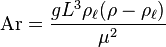$\mathrm{Ar} = \frac{g L^3 \rho_\ell (\rho - \rho_\ell)}{\mu^2}$

where:

•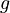$g$ is the local external field (for example gravitational acceleration), m/s2,
•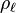$\rho_\ell$ is the density of the fluid, kg/m3,
•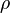$\rho$ is the density of the body, kg/m3,
•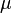$\mu$ is the dynamic viscosity, kg/ms,
•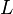$L$ is the characteristic length of body, m.In Spanish: Número de Arquímedes para niños